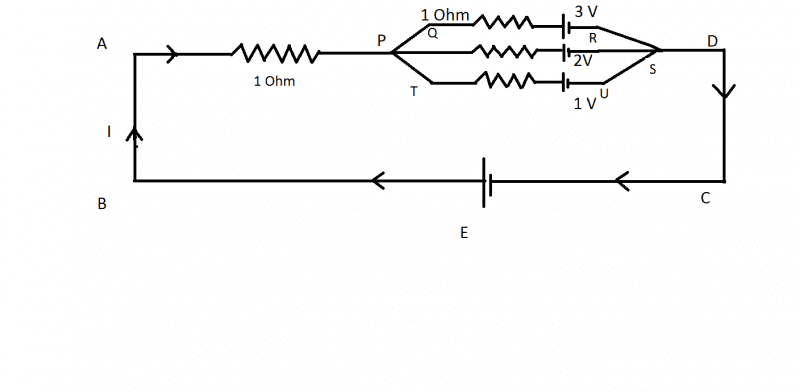# Kirchhoff's Loop Rule Problem.

Prashasti

## Homework Statement

Actually, the main question is to find out the potential difference between the points 'A' and 'D'.
The cell with emf 'E' has not been shown in the main question, but I have assumed it to be connected between 'A' and 'D'. Each resistor is of 1 Ohm.

2. The attempt at a solution
While solving the question, I applied Kirchhoff's Laws
Let 'I' be the current and let 'I1' be the current through the branch QR.
Since the total resistance offered by the branches (QR, PS, TU) is same (One ohm) , the currents through them will also be the same.
Assuming E > 3 V ( where 'E' is the emf of the cell)

Applying Kirchhoff's Current Law,
I = 3 I1
Applying KVL to the loop BAPQRDCB,
Δ V = 0
So, I(1) + I1(1) + 3 = E ....(1)
Similarly,
When KVL is applied to the loop BAPSDCB,
I(1) + I1 (1) + 2 = E ....(2)
(1) = (2), as E = E,
Which, on solving, gives 2 = 3!
Where did I go wrong?Last edited:

Homework Helper

## Homework Statement

Actually, the main question is to find out the potential difference between the points 'A' and 'D'.
The cell with emf 'E' has not been shown in the main question, but I have assumed it to be connected between 'A' and 'D'. Each resistor is of 1 Ohm.

2. The attempt at a solution
While solving the question, I applied Kirchhoff's Laws
Let 'I' be the current and let 'I1' be the current through the branch QR.
Since the total resistance offered by the branches (QR, PS, TU) is same (One ohm) , the currents through them will also be the same.

That is not true. The potential difference is the same across the three branches: VP-VS. As there are different cells in every branch, the voltages across the resistors are also different.

You can denote the currents flowing in the branches I1, (upper branch) I2 (middle branch) and I3 (bottom branch). Then I=I1+I2+I3. Write the loop equation for the loops which contain E and the series 1 ohm and one of the branches.Homework Helper
The cell with emf 'E' has not been shown in the main question, but I have assumed it to be connected between 'A' and 'D'.
1) What was shown in the main question ? Nothing there? Or a short-circuit ? Was anything mentioned about E at all ?

Since the total resistance offered by the branches (QR, PS, TU) is same (One ohm) , the currents through them will also be the same.
No. There are voltage sources too !
2) so that gets rid of I = 3 I1. You will need to split up I in I1, I2, I3.

 ah, ehild was faster !

Prashasti
No, only the portion of the figure between the points A and D has been given.

But, I've assumed E > 3V.. So the current will pass according to the cell with emf 'E' only? Am I right?

Staff Emeritus
Homework Helper
Gold Member
No, only the portion of the figure between the points A and D has been given.

But, I've assumed E > 3V.. So the current will pass according to the cell with emf 'E' only? Am I right?
No. You are not correct about that.

Don't introduce anything extra into the circuit.

Homework Helper
Was the current I given? Or were you asked to give the potential difference in terms of I?

Prashasti
Was the current I given? Or were you asked to give the potential difference in terms of I?

Prashasti
No. You are not correct about that.

Don't introduce anything extra into the circuit.
What would have happened if there was a question with the same circuit diagram and a cell with emf (E) 12 V was present there?

Homework Helper
If it is no indication that current flows through the leftmost resistor, you have to assume it zero. There is current in the three branches only, and find the potential difference between points A and D.

•Prashasti
Staff Emeritus
•